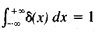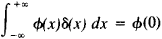# Delta Function

Also found in: Wikipedia.

## delta function

[′del·tə ‚fəŋk·shən]
(mathematics)
A distribution δ such that is ƒ(x). Also known as Dirac delta function; Dirac distribution; unit impulse.
McGraw-Hill Dictionary of Scientific & Technical Terms, 6E, Copyright © 2003 by The McGraw-Hill Companies, Inc.
The following article is from The Great Soviet Encyclopedia (1979). It might be outdated or ideologically biased.

## Delta Function

(also δ function, Dirac delta function, or δ(x)), a symbol used in mathematical physics in solving problems in which there are point magnitudes (for example, point load and point charge). The delta function can be defined as the density of the distribution of masses, for which a unit mass is concentrated at the point x = 0, while the mass at all other points is equal to zero. Therefore, it is assumed that δ(x) = 0, when x ≠ 0 and δ(0) = ∞, while(“infinite splash” of a “unit intensity”). More precisely, the delta function is the name of the generalized function defined by the equalitywhich is valid for all continuous functions φ(x).

In the theory of generalized functions, the delta function is the name of the functional itself, which is defined by this equality.

References in periodicals archive ?
Mathematically the Dirac delta function [delta](x) is discontinuous and has to be smoothed for numerical implementation:
Then scattering of relativistic fermions due to a single quaternionic Dirac delta function has been studied.
The conclusion is that the nonlinear coherent states are not full coherent although their P-representation is a delta function. Then, they do not satisfy the notion of classicalness introduced by Glauber in his quantum theory of optical coherence .
where f is a function of the position vector x and [delta] (x - x') is the Dirac delta function. Also, n is the volume of the integral that contains x.
We remember that every considering condition (5.8) is of the form (1.3) or, applying the popular notation [[integral].sup.1.sub.0] [u.sub.i](y)[delta](y - x) dy = [u.sub.i](x) for every component [u.sub.i] with the delta function, conditions (5.8) can be rewritten by
It consists of an opaque potential barrier with an oscillating delta function well-([f.sub.0] + [DELTA]f cos([omega]t))[delta](x - [x.sub.0]) in its center.
where [L.sub.P] is the number of the multipath components, [mathematical expression not reproducible] is the complex fading coefficient of the ith multipath component, [absolute value of [a.sub.i]] presents the amplitude, [[phi].sub.i] means the phase and obeys a uniform distribution U(0, 2[pi]) , [[tau].sub.i] denotes the time delay for the ith multipath component, and [delta] represents the Dirac delta function.
where [??](z) = [[integral].sub.x[greater than or equal to]0] M(x)[delta](z - <a, x>)dx and [delta](*) is the delta function.
Instead of the differentiated function (d[D.sub.i]/dx), this study used an approximate delta function, which is also known as normal distribution function.
In our endemic model, letting a =1 and h(t) = [delta](t - 1) where [delta] is the Dirac delta function, we note that each infection increases the force of the infectivity by one unit.
We considered the indeterminate beam problem shown in Figure 3, which is a fixed end supported beam applied by a Dirac delta function [12,13].

Site: Follow: Share:
Open / Close